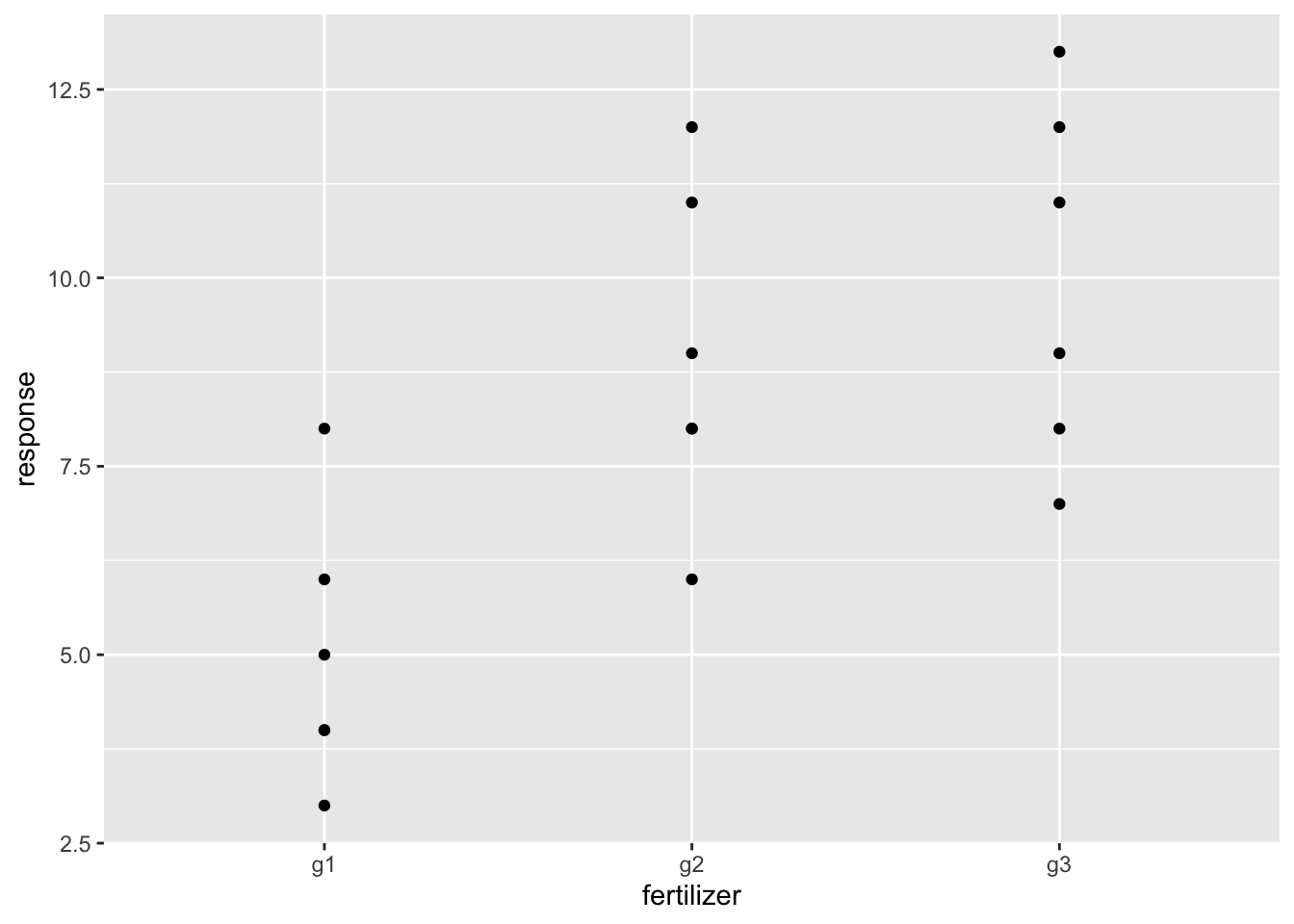We want to study the effect of 3 fertilizers in plant growth (example from wikipedia). So the factor fertilizer has 3 levels or groups.

library('plyr')
library('ggplot2')
g1<-c(6,8,4,5,3,4) #growth under fertilizer 1
g2<-c(8,12,9,11,6,8) #growth under fertilizer 2
g3<-c(13,9,11,8,7,12) #growth under fertilizer 3

d1<-data.frame(response=g1,fertilizer='g1')
d2<-data.frame(response=g2,fertilizer='g2')
d3<-data.frame(response=g3,fertilizer='g3')
dat<-rbind(d1,d2,d3)
dat
##    response fertilizer
## 1         6         g1
## 2         8         g1
## 3         4         g1
## 4         5         g1
## 5         3         g1
## 6         4         g1
## 7         8         g2
## 8        12         g2
## 9         9         g2
## 10       11         g2
## 11        6         g2
## 12        8         g2
## 13       13         g3
## 14        9         g3
## 15       11         g3
## 16        8         g3
## 17        7         g3
## 18       12         g3

We plot the data

ggplot(data=dat,aes(x=fertilizer,y=response))+geom_point()## Manual calculation

### Variations: sums of squares

The variation or sum of squares between groups SSB is

overallMean<-mean(dat$response) overallMean ##  8 SSBforEachGroup<-ddply(dat,.(fertilizer),function(d) { m<-mean(d$response)
ss<-length(d$response)*(m-overallMean)^2 data.frame(ss) }) SSBforEachGroup ## fertilizer ss ## 1 g1 54 ## 2 g2 6 ## 3 g3 24 SSB<-sum(SSBforEachGroup$ss)
SSB
##  84

The sum of squares within each group SSW is

SSWforEachGroup<-ddply(dat,.(fertilizer),function(d) {
m<-mean(d$response) ss<-sum((d$response-m)^2)
data.frame(ss)
})
SSWforEachGroup
##   fertilizer ss
## 1         g1 16
## 2         g2 24
## 3         g3 28
SSW<-sum(SSWforEachGroup$ss) SSW ##  68 The total sum of squares SST is SST<-sum((dat$response-overallMean)^2)
SST
##  152

which is equal to

SSB+SSW
##  152

### Degrees of freedom

The degrees of freedom associated to SSB is the number of groups minus 1

dfSSB<-length(unique(dat$fertilizer))-1 dfSSB ##  2 The degrees of freedom associated to SSW is the number of measures minus the number of groups dfSSW<-length(dat$response)-length(unique(dat\$fertilizer))
dfSSW
##  15

### Normalized sums of squares

NSSB<-SSB/dfSSB
NSSB
##  42
NSSW<-SSW/dfSSW
NSSW
##  4.533333

### F

f<-NSSB/NSSW
f
##  9.264706

The critical value that F must exceed to reject the null hypothesis (there is not effect of the fertilizer on response growth) which a probability of 5% that we reject the null hypothesis when is true is

fCritical<-qf(.95,dfSSB,dfSSW) #quantile function
fCritical
##  3.68232

We reject the null hypothesis as

f>fCritical
##  TRUE

### p

We can calculate which is the probability that we reject the null hypothesis when is true given our F

1-pf(f,dfSSB,dfSSW)
##  0.002398777

We can plot the distribution of F for dfSSB and dfSSW degrees of freedom, our F and Fcritical

fseq<-seq(0,10,.1)
fDis<-data.frame(fseq,p=df(fseq,dfSSB,dfSSW))
fDisCritical<-subset(fDis,fseq<fCritical)
ggplot(data=fDis,aes(x=fseq,y=p,color='distribution'))+geom_line()+
geom_vline(aes(xintercept=f,color='our F'))+
geom_ribbon(data=fDisCritical,aes(x=fseq,ymin=0,ymax=p,fill='F less than critical value'))+
theme(legend.position='none')## Direct calculation

oneway<-aov(response~fertilizer,data=dat)
summary(oneway)
##             Df Sum Sq Mean Sq F value Pr(>F)
## fertilizer   2     84   42.00   9.265 0.0024 **
## Residuals   15     68    4.53
## ---
## Signif. codes:  0 '***' 0.001 '**' 0.01 '*' 0.05 '.' 0.1 ' ' 1

The first column are the degrees of freedom, the second is the sum of squares, the third are the normalized sum of squares. For these columns the first row corresponds to the variation between groups (fertilizer) and the second row to the variation within groups (Residuals).

The fouth column if the F, and the fifth column is the probabilitythat we reject the null hypothesis when is true given our F.

The same result can be obtained using lm

linModel<-lm(response~fertilizer,data=dat)
anova(linModel)
## Analysis of Variance Table
##
## Response: response
##            Df Sum Sq Mean Sq F value   Pr(>F)
## fertilizer  2     84  42.000  9.2647 0.002399 **
## Residuals  15     68   4.533
## ---
## Signif. codes:  0 '***' 0.001 '**' 0.01 '*' 0.05 '.' 0.1 ' ' 1